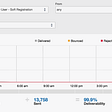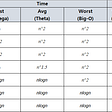# What is an algorithm?

Algorithms are not just found in computer programs or applications, they are an unavoidable aspect of our life as well. Algorithm is a set of instructions that need to be performed for a task to be done. It can also be viewed as a tool for solving a well-defined computational problem.

This can either be a simple process, such as multiplying two numbers, or a complex algorithm such as the ones used in large-scale applications. They provide aid to our lives as it gives a step-by-step solution to any problem that needs to be solved. Scientific achievements in various areas have been made by the use of algorithms, that make task completion easier as well as faster. It is majorly used for processes such as searching, sorting, graph analysis, etc. Here I will discuss some of the basic sorting algorithms that are used in computer programs.

To answer this question, one doesn't need to be a computer scientist! Sorting helps things to be arranged in an orderly or organized manner. It makes searching for things easier. For example, let us take a real-life example of bookshelves in a library. If all the books in the library are arranged according to their genres, it becomes easier for anyone to search for the book that they want. They just simply have to go to the shelf that contains books of their preferred genre and get the book from there. It simply saves their time, as they won't have to search in hundreds of other books to get their preferred book.

Similarly, in the world of computers, there is a lot of data that needs to be sorted. Computers use various sorting algorithms such as merge sort, quick sort, insertion sort, etc. to have the data sorted. Out of all the sorting algorithms, two algorithms, merge sort and bubble sort have been discussed here.

# Merge sort

Merge sort is a comparison-based sorting algorithm that is based on the divide and conquers algorithm. As the name suggests, It divides the array into halves until we reach the base case of the array that is with 1 element. After this division of the original input array takes place, the merge sort function takes the sorted sub-arrays and merges them to obtain the entire sorted array.

As this method uses the divide and conquer strategy, it sorts the array quickly as well as efficiently.

An image taken from https://www.programiz.com/dsa/merge-sort shows how the array is divided.

Given below is pseudocode for merge sort.(https://www.tutorialspoint.com/data_structures_algorithms/merge_sort_algorithm.htm)

procedure mergesort( var a as array )

if ( n == 1 ) return a

var l1 as array = a … a[n/2]

var l2 as array = a[n/2+1] … a[

l1 = mergesort( l1 )

l2 = mergesort( l2 )

return merge( l1, l2 )

end procedure

procedure merge( var a as array, var b as array )

var c as array

while ( a and b have elements )

if ( a > b )

add b to the end of c

remove b from b

else

add a to the end of c

remove a from a

end if

end while

while ( a has elements )

add a to the end of c

remove a from a

end while

while ( b has elements )

add b to the end of c

remove b from b

end while

return c

end procedure

Now as we have seen how merge sort works, let us now see a few advantages of merge sort:

1)Merge sort has a worst-case time complexity of O(nlogn). Making it a better choice over other sorting algorithms.

2)It is efficient for any size of data, be it large or small.

3)Merge sort is stable. Here the original order of the input set is preserved.

In addition to this, merge sort is one of the best sorting algorithms for sorting a linked list. In this case, implementation of merge sort is comparatively easy by implementing it in such a way that it requires only Θ(1) extra space. Sorting algorithms such as quicksort are not efficient in the case of linked lists, and others such as heapsort completely impossible.

# Bubble sort

Unlike merge sort, Bubble sort compares two elements and swaps them if they are not in the right order(ascending or descending). The process is repeated until the array is sorted. Now, the problem with bubble sort is that it is slow and its worst case, and average time complexity is O(n²). Due to this, bubble sort loses its practical utility. However, bubble sort has a time complexity of O(n) when the array is already sorted, making it advantageous to be used in the case of an already sorted array. It also performs well if the array needs a lesser number of swaps. But, bubble sort should be avoided while dealing with large data, such as in real-life applications.

image bubble sort(faceprep.in)

Given below is a pseudocode for the implementation of bubble sort. It is going to repeatedly check for two elements and swap them if they are in the wrong order until the array is completely sorted.

`procedure bubbleSort(A : list of sortable items)n := length(A)repeatswapped := falsefor i := 1 to n-1 inclusive do/* if this pair is out of order */if A[i-1] > A[i] then/* swap them and remember something changed */swap(A[i-1], A[i])swapped := trueend ifend foruntil not swappedend procedure`

# Conclusion

Here I have discussed two of the most common sorting algorithms, i.e, merge sort and bubble sort. Both have their own advantages and disadvantages and differ widely in terms of practicality. However, just by looking at the time complexity, we cannot conclude which algorithm is the best sorting algorithm. Even though sorting may seem like a simple concept, but efficient sorting is critical when dealing with large amounts of data. Sorting is the basis for more complex computer programs such as searching for files on a computer, finding the shortest route to a destination, and compressing data. Each sorting algorithm performs more efficiently according to the given situation.

--

--

--

Love podcasts or audiobooks? Learn on the go with our new app.

## Announcement: Hashpay Wallet v3.1.0 Update## What Are Proper Use Cases For Snapshot Testing React Components?## 3 Lessons Learned From My Passing My AWS Solutions Architect Associate Exam## Automating the clinical coding process in an NHS Trust — ep. 3## LEARN TO CODE | BTC## When emails attack — how we accidentally spammed our own users## More from Medium